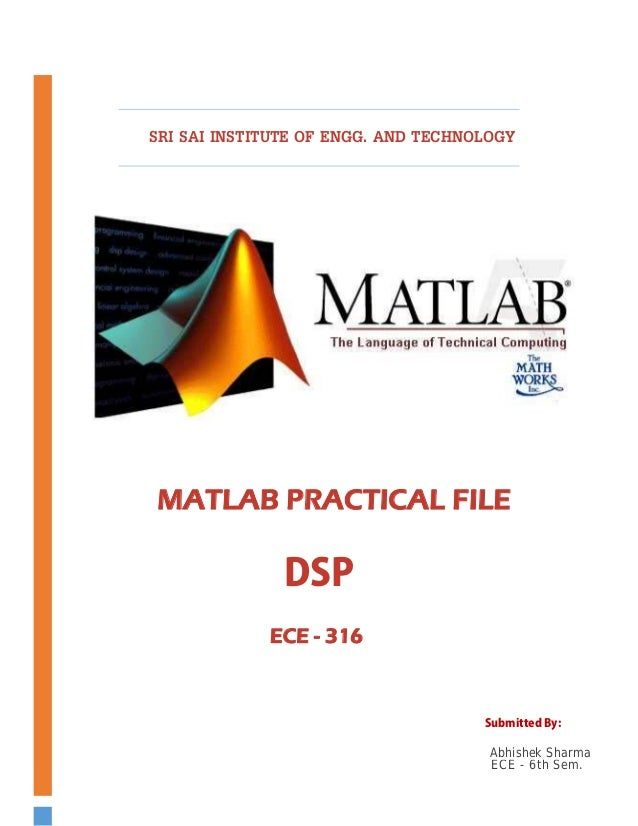Successfully reported this slideshow.
We use your LinkedIn profile and activity data to personalize ads and to show you more relevant ads. You can change your ad preferences anytime.Upcoming SlideShare
Loading in …5
×

# MATLAB Programs For Beginners. | Abhi Sharma

This is MATLAB's 10 most easy & most basic programs that I's supposed to submit in my practicals. In this document I've complied 10 MATLAB programs from basic to advanced through intermediate levels, But overall they are for beginners only. It's only a 26 pages doc. for academic purposes. well, What else a student can offer you, huh? LOLz

• Full Name
Comment goes here.

Are you sure you want to Yes No
Your message goes here• Login to see the comments

### MATLAB Programs For Beginners. | Abhi Sharma

1. 1.        SRI SAI INSTITUTE OF ENGG. AND TECHNOLOGY                  MATLAB PRACTICAL FILE  DSP ECE - 316  Submitted By:  Abhishek Sharma ECE - 6th Sem.
2. 2. TABLE OF CONTENTS ContentsInroduction To MATLAB _____________________________________________________________________ 1 Program For Impulse Function _____________________________________________________________ 4 Program For Unit Step Function ____________________________________________________________ 6 Program For Unit Ramp Function __________________________________________________________ 8 Program For Exponential Function ______________________________________________________ 10 Program For Real Value Function ________________________________________________________ 16 Program For Shifting Function ___________________________________________________________ 14 _Program For Addition Function ___________________________________________________________ 16 Program For Multiplication Function ____________________________________________________ 18 Program For Convolution Function ______________________________________________________ 20 Program For Folding Function ____________________________________________________________ 23
3. 3. WHAT IS MATLAB? “AN INTRODUCTION”   • It stands for MATrix LAbORATORY • It is developed by The Mathworks Inc. • It is an interactive, integrated, environment • For numerical computations • For symbolic computations • For scientific visualizations • It is a high level programming language • Program runs in interpreted, as opposed to compiled, mode • MATLAB is a high level technical computing language and interactive environment for algorithm development, data visualization, data analysis and numeric computation. Using the MATLAB product, you can solve technical computing problems faster than the traditional programming languages such as C, C++ and FORTRAN. • You can use MATLAB in a wide range of applications, including signal and image processing, communication, control design, test and measurement, financial modeling and analysis, and computational biology. Add on toolboxes(collection of special purpose MATLAB functions, available separately) extend the MATLAB environment to solve particular classes of problems in these application areas. • MATLAB provides a number of features for documenting and sharing your work. You can integrate your MATLAB code with other languages and applications, and distribute your MATLAB algorithms and applications.          1
4. 4. Characterstics Of MATLAB:  • Programming Language Based(principally) On Matrices. • Slow compared with FORTRAN or C because it is an interpreted language, i.e not pre‐ compiled. Avoid for loops, instead use vector form whenever possible. • Automatic memory management, i.e you don’t have to declare arrays in advance. • Intuitive, easy to use. • Compact (array handling is Fortran 90‐like). • Shorter program development time than traditional programming languages such as FORTRAN and C. • Can be converted into C code via MATLAB compiler for better efficiency. • Many applications‐ specific toolboxes available. • Coupled with Maple for symbolic computations. • On shared‐memory parallel computers such as the SGI Origin2000, certain operations processed in parallel autonomously when computation load warrants. KEY FEATURES:-  • High level language for technical computing. • Development environment for managing code, files, and data. • Interactive tools for iterative exploration, design and problem solving. • Mathematical functions for linear algebra, statistics, Fourier analysis, filtering, optimization, and numerical integration • 2‐D and 3‐D graphical functions for visualizing data. • Tools for building custom graphical user interfaces. • Functions for integrating MATLAB based algorithm with external application and languages, such as C, C++, FORTRAN, Java, and Microsoft Excel.       2
5. 5. EXAMPLES:-  • Matrix computation and linear algebra. • Solving nonlinear equation. • Numerical solution of differential equation. • Mathematical optimization. • Statistical and data analysis. • Signal Processing. • Modeling of dynamical systems. • Solving partial differential equation. • Simulation of Engg. Systems. USES IN ENGG. COMPANIES:‐   • Numerical analysis • Signal and system. • Modeling of dynamical systems. • Automatic control. BASIC COURSES:‐   • Automatic control advanced course. • Hybrid and embedded. • Control system. • Chemical process control. • Control process control. • Signal theory. • Digital signal processing. • Adaptive signal processing. • Signal processing project. • Communication theory. • Advance communication theory.   3
6. 6. Program - 1 To Develop Elementary Signal For Impulse Function  Program:  a=[‐2;1;2] b=[zeros(1,2),ones(1,1),zeros(1,2)] stem(a,b) xlabel(‘a‐‐‐‐>’) ylabel(‘amp‐‐‐>’)   Result:  a= ‐2   ‐1    0    1    2  b= 0    0    1    0    0        4
7. 7. Graph For Impulse Function:               5
8. 8. Program - 2 To Develop Elementary Signal For Unit Step Function  Program:  n=input(’enter the value of n’) a=[1:1:n] b=[ones,n] subplotes stem(a,b) xlabel(‘n…..>’) ylabel(‘amplitude’)   Result of unit step function:  Enter the value of n n=5 a=0  1  2  3  4 b= 1   1   1   1   1                                                                                                        6
9. 9. Graph For Unit Step Function:  7
10. 10. Program - 3  To Develop Elementary Signal For Unit Ramp Function  Program:  a=[2:1:8] b=[0;1;6] subplot stem(a,b) xlabel(‘n.’) ylabel(‘amp….’)   Result of unit ramp function:  a=2    3    4    5    6    7    8  b= 0    1    2    3    4    5    6          8
11. 11. Graph For Unit Ramp Function:  9
12. 12. Program - 4 To Develop Exponential Function Of (Given) Sequence  Program:  n=input(‘enter the value of n’) a=input(‘enter the value of a’) t=[0:1:n] y=exp(a*t) subplot stem(t,y) xlabel(‘a’) ylabel(‘n’)  Result of exponential: Enter the value of n10 n= 10 enter the value of a0.5 a= 0.5000 t=0   1   2   3   4   5   6   7   8   9   10 y=columns 1 through 10 1.0000  1.6487  2.7183  4.4817  7.3891  12.1825  20.0855  33.1155  54.5982  90.0171 Column11 148.4132  10
13. 13. Graph For Exponential Function:  11
14. 14. Program - 5 To Develop Elementary Signal For Real Value  Program:  n=[0,1,2,3,4,5] a=[0.5] y=a.^n subplot stem(n,y) xlabel(‘n…..’) ylabel(‘a’)  Result of Real Value No.:  n= 0   1   2   3   4   5    a= 0.5000  y = 1.0000   0.5000   0.2500   0.1250   0.0625   0.0313    12
15. 15. Graph For Real Value Function: 13
16. 16. Program - 6 To Develop Elementary Signal For Shifting Program:  a=[‐3:1:3] b=[1.2.3.2.1.1.2] subplot(3,1,1) stem(a,b) xlabel(‘n‐‐‐‐>’) ylabel(‘amp‐‐‐>’) a=‐a subplot(3,1,2) stem(a,b) xlabel(‘n‐‐‐‐>’) ylabel(‘amp‐‐‐>’)  Result:  a = ‐3   ‐2   ‐1   0   1   2   3 b = 1   2   3   2   1   1   2 a = 3   2   1   0   ‐1   ‐2   ‐3    14
17. 17.  Graph For Shifting Function:               15
18. 18.   Program - 7 To Develop Elementary Signal For Addition Of Two  Sequences  Program:  n=[‐3:1:3] b=[2,3,0,1,3,2,1] subplot(5,1,1) stem(n,b) xlabel(n….>)    ylabel(amplitude) title(input of signal b) a=[3,4,5,6,7,8,9] subplot(5,1,3) stem(n,b) ylabel(amplitude) title(input of signal a) z=b+a subplot(5,1,5) stem(n,a) xlabel(n….>)    ylabel(amplitude) title(addition of two signal is z(n)) Result of Addition:  2   3   0   1   3   2   1  a = 3   4   5   6   7   8   9  z = 5   7   5   7   10   10   10  16
19. 19. Graph For Addition Function:                                                                                             17
20. 20. Program - 8 To Develop Elementary Signal For Multiplication Of Two  Sequences  Program:  n=[‐2:1:3] x=[1,2,3,4,5,6] subplot(3,1,1) stem(n,x) xlabel(n‐‐‐‐>) ylabel(amp‐‐‐>) y= z=(x*y) subplot(3,1,2) stem(n,z) xlabel(n‐‐‐‐>) ylabel(amp‐‐‐>) Result:  n = ‐2   ‐1   0   1   2   3 x = 1   2   3   4   5   6 y = 2 z = 2   4   6   8   10   12  18
21. 21. Graph For Multiplication Function:  19
22. 22. Program - 9 To Develop The Elementary Signal For Convolution Of  Two Sequences  Program:  X=input(‘enter the value of x’) h=input(‘enter the value of h’) y=conv(x,h) subplot(3,1,1) stem(x) xlabel(‘n….>’)    ylabel(‘amplitude….>’) subplot(3,1,2) stem(h) xlabel(‘n….>’)    ylabel(‘amplitude….>’) subplot(3,1,3) stem(y) xlabel(‘n….>’)    ylabel(‘amplitude….>’)    20
23. 23. Result of convolution:  Enter the sequence of x[1,2] X=1   2 Enter the sequence of h[1,2,3,4]  h = 1   2   3   4 y = 1   4   7   10   8                      21
24. 24. Graph For Convolution Function:              22
25. 25. Program - 10 To Develop Elementary Signal For Folding  Program:  a=[‐3:1:3] b=[1,2,3,2,1,1,2] subplot(3,1,1) stem(a,b) xlabel(‘n….. >’) ylabel(‘amp…..>’) a= ‐a subplot(3,1,2) stem(a,b) xlabel(‘n…..>’) ylabel(‘amp…..>’)  Result of Folding:  a= ‐3   ‐2   ‐1   0    1    2    3 b=  1    2    3    2    1    1     2 a=  3    2    1    0   ‐1   ‐2   ‐3     23
26. 26. Graph For Folding Function:               24